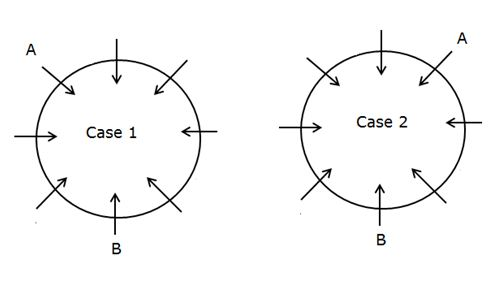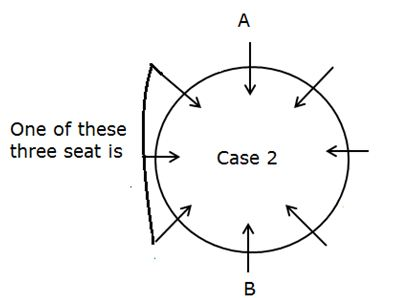# Confusing Statements in Seating Arrangement – SBI PO 2019

Hi IGians,

As SBI PO preliminary examination is around the corner and students must have started their preparation immediately after the release of its notification. Last year there are a few changes introduced in mains examination but there is no major change in prelims.

Students are frequently got confused in some statement in Puzzle and seating arrangement. Here we have given the clear idea about the most confusing statements in seating arrangements.

Confusing statement:

Confusing statement – 1: B sits two places away from A.

Either B sits second to the right of A or second to the left of A. It is also same for circular, Square , Rectangular , Parallel row,  Hexagonal and any other seating arrangement.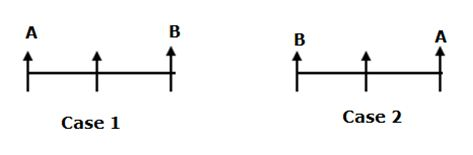Similarly:

Three places away from A four or five means this same logic is applied.

Confusing statement – 2: For circular Arrangement

If it is given that

Circumference of circular table = 240m, then it is given as eight people facing center then the distance between adjacent person becomes 240/8 = 30m.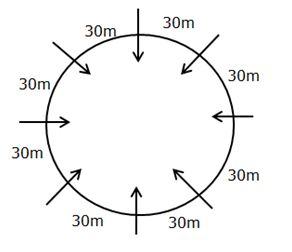• In this given that A is 30m away from B means Either A sits immediate right or immediate left of B.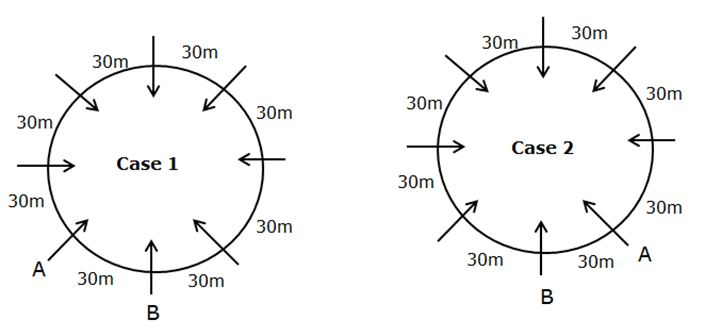• If A is 90m away from is means, A is third to the right or third to the left of B.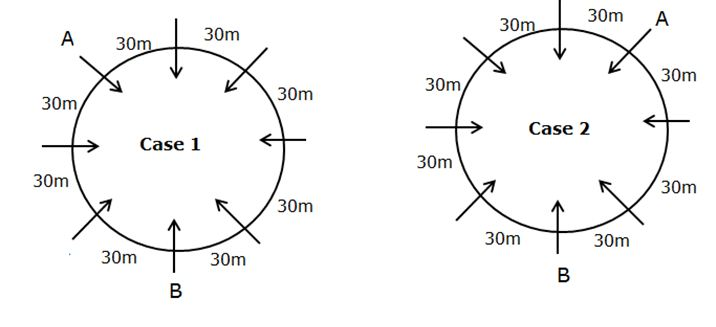Similarly:

If the radius is given then we need to find out the circumference of the circle. By using the formula = 2πr. Then the circumference is divided by number of persons given in the question.

Confusing statement-3:

If perimeter is given then perimeter is divided by number of person given. If 8 person is given then the perimeter is divided by 8 or the distance between the adjacent person is equal to side of a square(a) divided by 2 or a/2.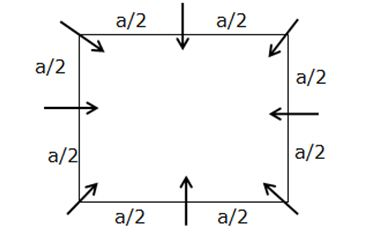Similarly for Rectangle:

Perimeter of square = 4a

Perimeter of rectangle = 2(l+b)

Confusing statement –  4 :

For linear row it is given that,

• Integral multiple of 5,
• Integral multiple of 6,
• Integral multiple of 7,
• Integral multiple of 4,

What does it means?

• Integral multiple means quantity multiplied by an integer.

Example: 8 people seated in a linear, row facing North. The distance between two people is integral multiple of 4 and the distance is increased from left to right.

It means,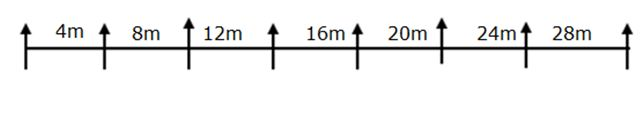Confusing statement – 5 :

• Number of persons sitting between A and B is same as number of person sitting between X and Y.If there are two person sits between A and B then same number of person sits between X and Y. Any type of seating arrangement it is applicable.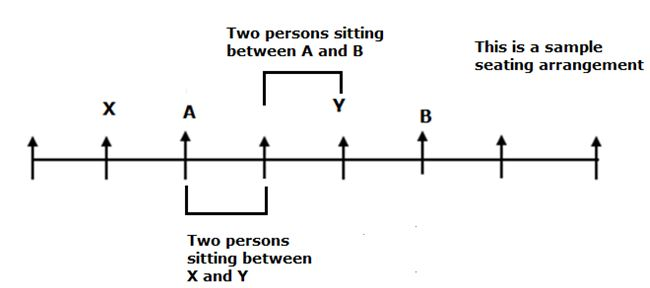Confusing statement- 6:

• Number of person sits between A and B is one more than that of number of person sits between X and Y. If there are Three person sits between A and B then number of person sits between X and Y is two.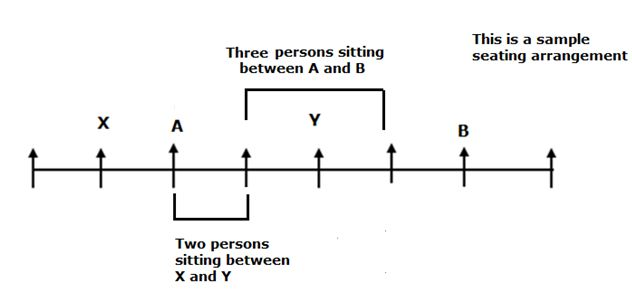Similarly for two more than,  three more than, etc..,

Confusing statement- 7:

• Number of person sits between A and B is one less than that of number of person sits between X and Y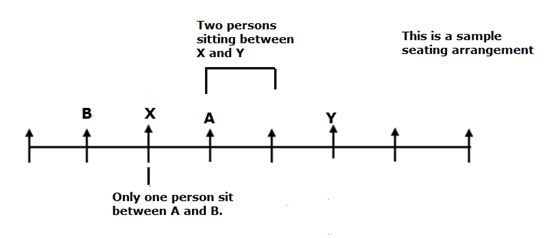Similarly, for two less than three less than etc…,

Confusing statement -8:

• If given that two concentric circle then you see the statement, B sits ahead of A or B sits in front of A it can be represent by,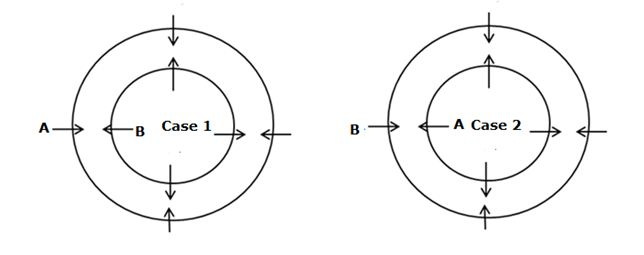Confusing statement -9:

For linear arrangement:

• At least two person sits to the left of A

If it is north facing row, then it can be represent by,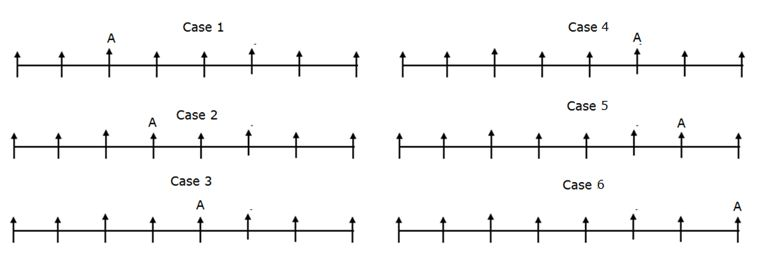Confusing statement- 10:

• Not more than three person sits to the right of A.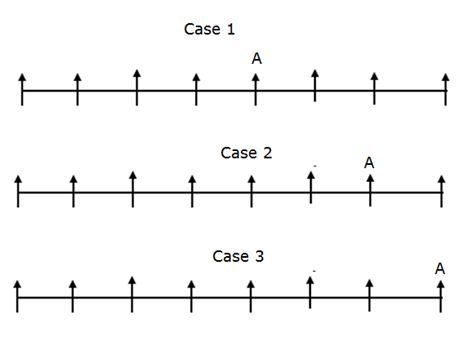Confusing statement -11:

• At most two person sits to the left of A. It can be represent by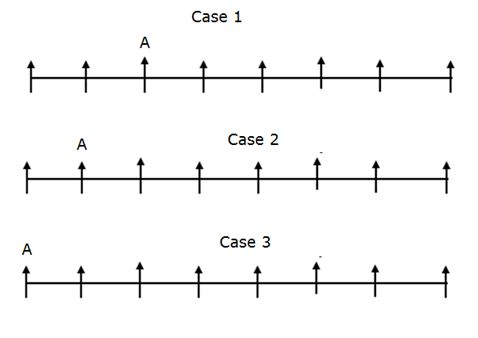Confusing statement -12:

• Not more than three person sits between A and B. It can be represent by,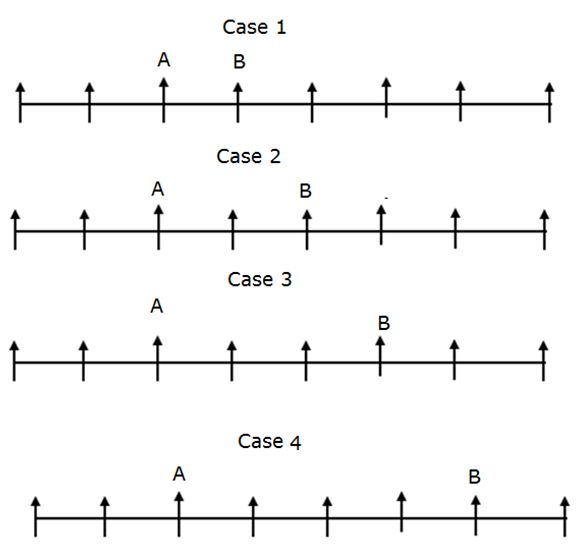• Not Less than three person sits between A and B. It means Number of sit between A and B is greater than or equal to 3.

Confusing statement- 13:

• A sits between B and C.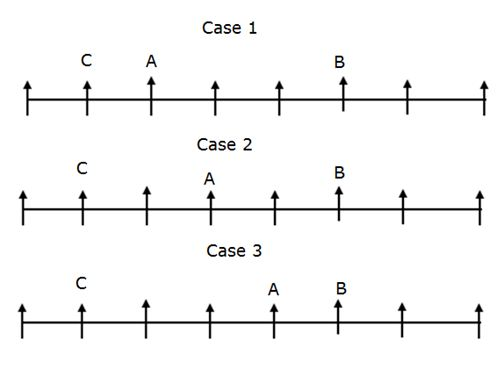Confusing statement -14:

• A sits exactly between B and C.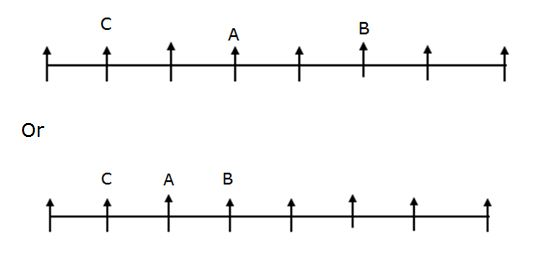Confusing statement -15:

• A sits exactly between B and C when counted from left of B.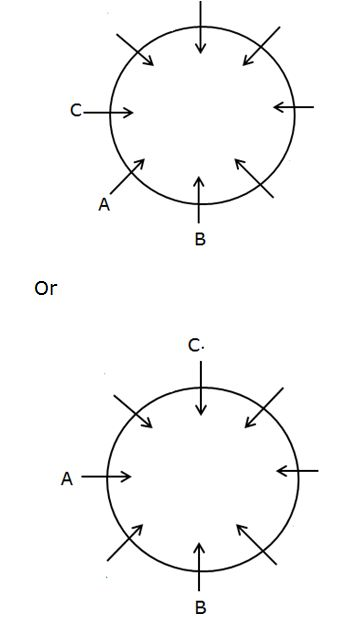Confusing statement -16:

In vacant seating arrangement,

For linear row:

• Two person sits between A and B means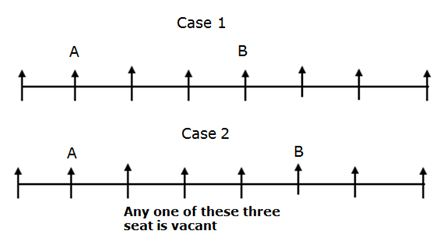• It is also for circular and square arrangement.